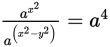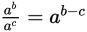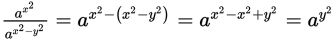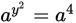# SAT Math Multiple Choice Question 880: Answer and Explanation

### Test Information

Question: 880

10. Ifand y > 0, what is the value of y?

• A. 0
• B. 1
• C. 2
• D. 4

Explanation:

C

Difficulty: Medium

Category: Passport to Advanced Math / Exponents

Strategic Advice: You can always rely on the rules of exponents, no matter how strange the exponents may look. Follow the rule for dividing powers of like bases:.

Getting to the Answer: Although the left side of the equation seems strange, it melts away once you apply the exponent rule:So, which means that y2 = 4 and y = 2 or -2. The question states that y > 0, so y must be 2, which is (C).